Include Top

# Overview of Basic Design of Experiments (DOE) Templates

The DOE templates are similar to the other SigmaXL templates: simply enter the inputs and resulting outputs are produced immediately. The DOE templates provide common 2-level designs for 2 to 5 factors. These basic templates are ideal for training, but use SigmaXL > Design of Experiments > 2-Level Factorial/Screening Designs to accommodate up to 19 factors with randomization, replication and blocking.

Click SigmaXL > Design of Experiments > Basic DOE Templates to access these templates:

• Two-Factor, 4-Run, Full-Factorial
• Three-Factor, 4-Run, Half-Fraction, Res III
• Three-Factor, 8-Run, Full-Factorial
• Four-Factor, 8-Run, Half-Fraction, Res IV
• Four-Factor, 16-Run, Full-Factorial
• Five-Factor, 8-Run, Quarter-Fraction, Res III
• Five-Factor, 16-Run, Half-Fraction, Res V

After entering the template data, main effects and interaction plots may be created by clicking SigmaXL > Basic DOE Templates > Main Effects & Interaction Plots. The DOE template must be the active worksheet.

DOE Templates are protected worksheets by default, but this may be modified by clicking SigmaXL > Help > Unprotect Worksheet.

Advanced analysis is available, but this requires that you unprotect the DOE worksheet. The following example shows how to use Excel’s Equation Solver and SigmaXL’s Multiple Regression in conjunction with a DOE template. Caution: If you unprotect the worksheet, do not change the worksheet title (e.g. Three-Factor, Two-Level, 8-Run, Full-Factorial Design of Experiments). This title is used by the Main Effects & Interaction Plots to determine appropriate analysis. Also, do not modify any cells with formulas.

## Three Factor Full Factorial Example Using DOE Template

1. Open the file DOE Example - Robust Cake.xlsx. This is a Robust Cake Experiment adapted from the Video Designing Industrial Experiments, by Box, Bisgaard and Fung.
2. The response is Taste Score (on a scale of 1-7 where 1 is "awful" and 7 is "delicious").
3. The five Outer Array Reps have different Cooking Time and Temperature Conditions.
4. The goal is to Maximize Mean and Minimize StDev of the Taste Score.
5. The X factors are Flour, Butter, and Egg. Actual low and high settings are not given in the video, so we will use coded -1 and +1 values. We are looking for a combination of Flour, Butter, and Egg that will not only taste good, but consistently taste good over a wide range of Cooking Time and Temperature conditions.
6. Scroll down to view the Pareto of Abs. Coefficients for Average (Y).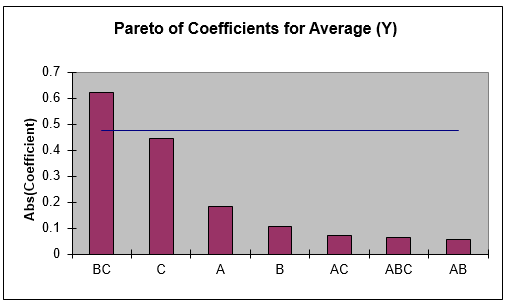7. The BC (Butter * Egg) interaction is clearly the dominant factor. The bars above the 95% confidence blue line indicate the factors that are statistically significant; in this case only BC is significant. Keep in mind that this is an initial analysis. Later, we will show how to do a more powerful Multiple Regression analysis on this data. (Also, the Rule of Hierarchy states that if an interaction is significant, we must include the main effects in the model used.)
8. The significant BC interaction is also highlighted in red in the table of Effects and Coefficients: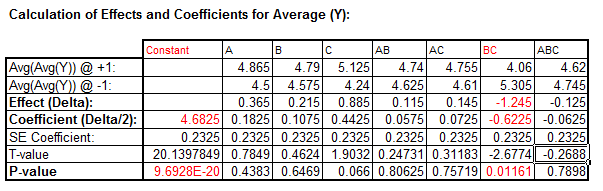9. The R-Square value is given as 27%. This is very poor for a Designed Experiment. Typically, we would like to see a minimum of 50%, with > 80% desirable.The reason for the poor R-square value is the wide range of values over the Cooking Temperature and Time conditions. In a robust experiment like this, it is more appropriate to analyze the mean response as an individual value rather than as five replicate values. The Standard Deviation as a separate response will also be of interest.

10. If the Responses are replicated, SigmaXL draws the blue line on the Pareto Chart using an estimate of experimental error from the replicates. If there are no replicates, an estimate called Lenth’s Pseudo Standard Error is used.
11. If the 95% Confidence line for coefficients were to be drawn using Lenth’s method, the value would be 0.409 as given in the table: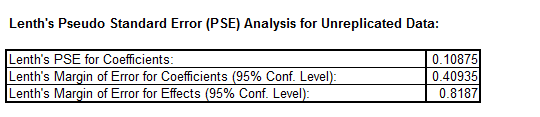This would show factor C as significant.

12. Scroll down to view the Pareto of Coefficients for StdDev(Y).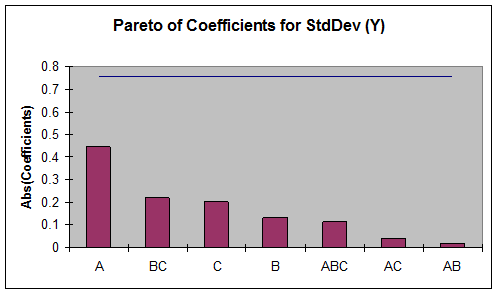13. The A (Flour) main effect is clearly the dominant factor, but it does not initially appear to be statistically significant (based on Lenth’s method). Later, we will show how to do a more powerful Regression analysis on this data.
14. The Pareto chart is a powerful tool to display the relative importance of the main effects and interactions, but it does not tell us about the direction of influence. To see this, we must look at the main effects and interaction plots. Click SigmaXL > Basic DOE Templates > Main Effects & Interaction Plots. The resulting plots are shown below: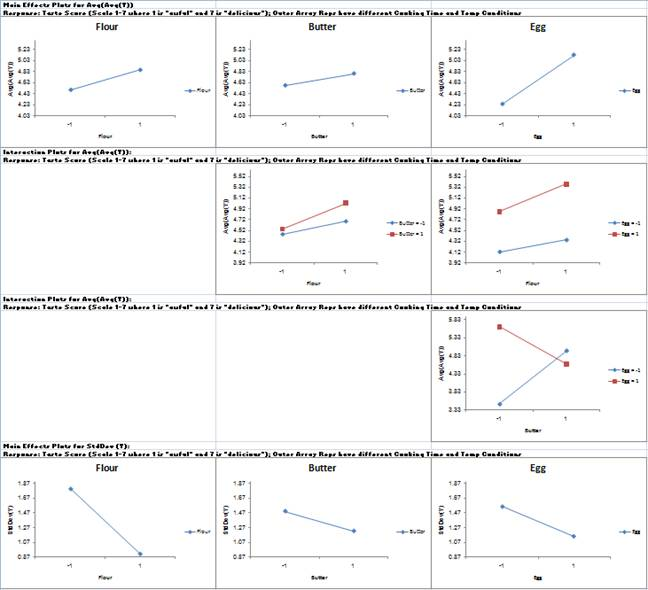15. The Butter*Egg two-factor interaction is very prominent here. Looking at only the Main Effects plots would lead us to conclude that the optimum settings to maximize the average taste score would be Butter = +1, and Egg = +1, but the interaction plot tells a very different story. The correct optimum settings to maximize the taste score is Butter = -1 and Egg = +1.
16. Since Flour was the most prominent factor in the Standard Deviation Pareto, looking at the Main Effects plots for StdDev, we would set Flour = +1 to minimize the variability in taste scores. The significance of this result will be demonstrated using Regression analysis.
17. Click on the Sheet Three-Factor 8-Run DOE. At the Predicted Output for Y, enter Flour = 1, Butter = -1, Egg = 1 as shown: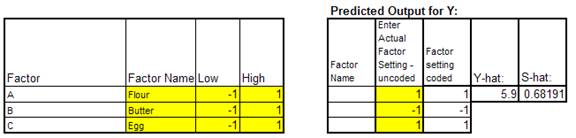The predicted average (Y-hat) taste score is 5.9 with a predicted standard deviation (S-hat) of 0.68. Note that this prediction equation includes all main effects, two-way interaction, and the three-way interaction.

### Multiple Regression and Excel Solver (Advanced Topics):

1. In order to run Multiple Regression analysis we will need to unprotect the worksheet. Click SigmaXL > Help > Unprotect Worksheet.
2. In the Coded Design Matrix, highlight columns A to ABC, and the calculated responses as shown:

3.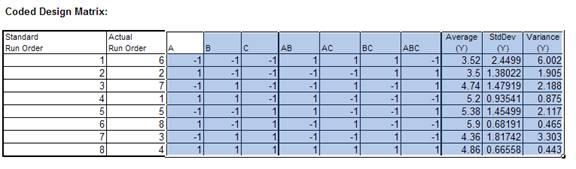4. Click SigmaXL > Statistical Tools > Regression > Multiple Regression. Click Next.
5. Select Average (Y), click Numeric Response (Y) >>; holding the CTRL key, select B, C, and BC; click Continuous Predictors (X) >> as shown:

6.7. Click OK. The resulting regression report is shown: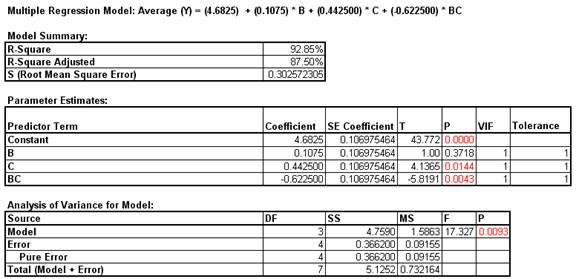Note that the R-square value of 92.85% is much higher than the earlier result of 27%. This is due to our modeling the mean response value rather than considering all data in the outer array. Note also that the C main effect now appears as significant.

8. Click on the Sheet Three-Factor 8-Run DOE.
9. With the Coded Design Matrix highlighted as before, click SigmaXL > Statistical Tools > Regression > Multiple Regression. Click Next.
10. Select StdDev (Y), click Numeric Response (Y) >>; select A, click Continuous Predictors (X) >> as shown:11. Click OK. The resulting regression report is shown: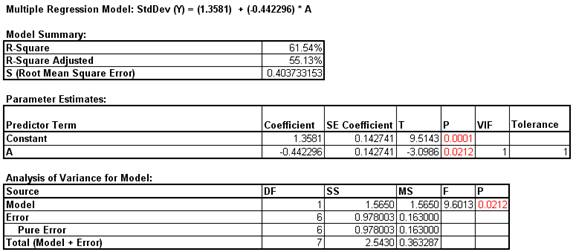Note that Factor A (Flour) now shows as a statistically significant factor affecting the Standard Deviation of Taste Score.

12. Now we will use Excel’s Equation Solver to verify the optimum settings determined using the Main Effects and Interaction Plots.
13. Click on the Sheet Three-Factor 8-Run DOE. At the Predicted Output for Y, enter 1 for Flour. We are setting this as a constraint, because Flour = +1 minimizes the Standard Deviation. Reset the Butter and Egg to 0 as shown: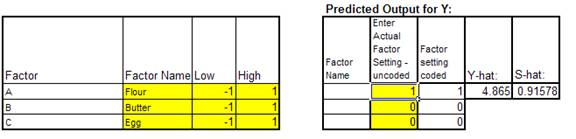14. Click Tools > Add-Ins. Ensure that the Solver Add-in is checked. If the Solver Add-in does not appear in the Add-ins available list, you will need to re-install Excel to include all add-ins.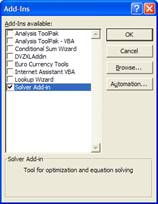15. Click OK. Click Tools > Solver. Set the Solver Parameters as shown: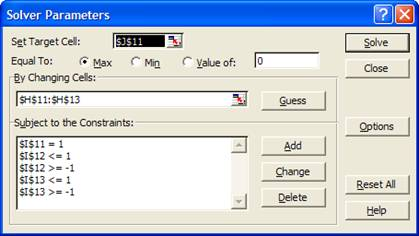Cell J11 is the Y-hat, predicted average taste score. Solver will try to maximize this value. Cells H11 to H13 are the Actual Factor Settings to be changed. Cells I11 to I13 are the Coded Factor settings where the following constraints are given: I11=1; I12 >= -1; I12 <= 1; I13 >= -1; I13 <=1.

16. Click Solve. The solver results are given in the Predicted Output for Y as Butter = -1 and Egg = 1.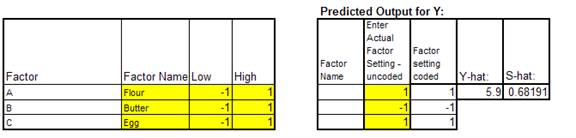17. Solver indicates that a solution is found: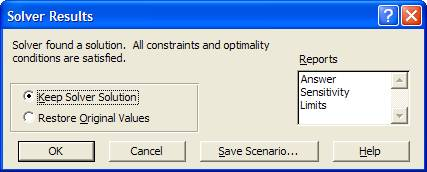18. Click OK to keep the solution.

# Web Demos

Our CTO and Co-Founder, John Noguera, regularly hosts free Web Demos featuring SigmaXL and DiscoverSim